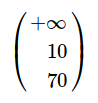# Displaying a text before a solution and a strange behaviour

1) Under Windows10

A=matrix(m,n,(0,1,1,0,6,18))
show($A =$,A)


This does not display with A= in latex style. How can I code what I expect ?

2) on my computer

%display latex
m=3 #nombre de contraintes
n=2 # nombre de variables
A=matrix(m,n,(0,1,1,0,6,18))
bmin=matrix(m,1,(12,0,0))
bmax=matrix(m,1,(oo,10,70))
c=matrix(1,n,(4.1,8))
show(A,bmin,bmax,c)
show(bmax)


10 and 70 are not displayed but sage substitute "$Apositivefinitenumber$"

edit retag close merge delete

Where, in which environment is missing the display (since i am starting sage either in a linux console, or in an eclipse / pycharm IDE)? And on which platform?

Sort by » oldest newest most voted

Hello, @Cyrille!

Concerning (1), remember: the best way to typeset a string as LaTeX output in Sage is using the LatexExpr function/class, instead of using a pair of $. So, in order to outputting A = as LaTeX, just do LatexExpr('A = '): show(LatexExpr('A ='), A)  Notice the matrix A itself doesn't need to be wrapped with a LatexExpr, since it is not a string. Concerning (2), matrices have rings associated to them, and Sage "coerces" (I believe that's the technical word) the ring to the most general one that fits the contents of the matrix. In your case, the presence of oo is making Sage assume your matrix should be associated with the The Infinity Ring, as you can see yourself if you write bmax.base_ring(). This ring assumes something is infinity ($+\infty$,$-\infty$or an unsigned$\infty$), zero or something finite. Unfortunately, this makes the ring almost blind to everything finite. For example, if you write InfinityRing(7) == InfinityRing(800)  you will get True, which shows you that this ring considers$7$and$800\$ the same.

Anyway, the solution is to specify another ring. In this case, you have to use SR (the symbolic ring):

bmax = matrix(SR, m, 1, (oo,10,70))


or

bmax = matrix(m, 1, (oo,10,70), ring=SR)


This will give you:WARNING: If you are trying to use the bmin and bmax arguments of add_constraint, I think you should stick to lists of values, instead of matrices. For example, you can use bmax = [oo, 10, 70] while working the Linear Problem, and once you solve it, you can use show(matrix(SR, m, 1, bmax)). Otherwise, the matrix ring seems to interfere with the constraint definition.

more